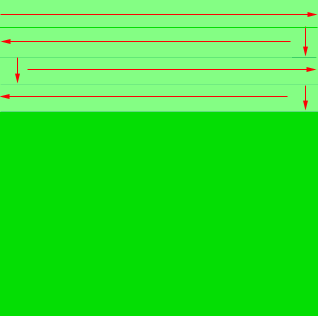If a man is mowing a 100' x 100' yard with a 20" push mower, how many miles has he walked when the yard is completely mowed? Ed level secondary Hi Ed. If we start with two assumptions, we can make good progress on this question: 1) pivoting 90 degree with the mower = 0 distance walked, 2) We can start anywhere and end anywhere Lets then say that the man starts at a corner and heads east. He mows the first strip of 20 inches for 100 feet, then pivots to the south. He mows for 20 inches (the same as the mower's width.He pivots to the west and mows 100 feet - the first 20 inches. When he gets to the end, he repeats the process.You can see from this that if you add the short square pieces at the ends (20 by 20) to the long row, you get the original 100 feet. If you multiply 100 feet by 12 you get the width of the yard in inches. Then divide by 20 inches to find the number of rows he mows. Each row is 100 feet long, so multiply by the row length. Add these up and you get the distance he has mowed (walked). This distance is in feet so you will need to divide by the number of feet in a mile to get the number of miles. This is a lower estimate since when you are mowing and reach the end of the row you turn the mower 90 degrees, mow the 20 inches, turn the mower 90 degrees again and the proceed. Each time you turn the mower you have to walk around a quarter circle to get behind the mower to proceed. The length of this arc you walk depends on the length of the handle. Hope this helps, Stephen and Penny